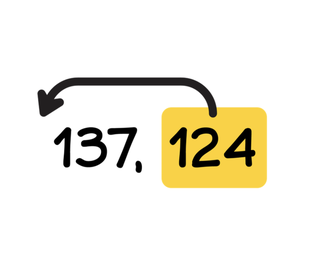Comparing and ordering numbers up to 1000

# Comparing and ordering numbers up to 1000

I can compare numbers from 100 to 1000 and put them in order.

No account needed.8,000 schools use Gynzy92,000 teachers use Gynzy1,600,000 students use Gynzy

## General

Student learn to compare the numbers from 100 to 1,000 and set them in correct numerical order. They also can determine when a number is more or less than a given number.

## Relevance

Discuss with students why it is important to be able to compare numbers and set them in the correct order. Say that you can use this to determine if you have enough money to buy something you want, like a bicycle.

## Introduction

The interactive whiteboard shows an amount that students must pay. Drag the bills to create the correct payment. Next the same amount will be shown in a HTO(hundreds, tens, ones) chart and on a number line. Explain what the H, T, and O stand for.

## Development

Show students the HTO(hundreds, tens, ones) chart and using the example show students that they can use the chart to compare numbers. By comparing the hundreds, tens and ones with each other, you discover which number is smallest, or the least, and which is biggest, or the most. Explain to students that you can also use the HTO-chart to determine the order of a set of numbers. Show this using an example. Next as a class compare a few numbers and set them in the correct order by dragging them to their correct locations on the interactive whiteboard.

To check that students understand comparing and ordering numbers to 1,000, you can ask the following questions/give the following exercises:
- When is a number more than another number?
- How do you know if a number is more or less than another number?
- Which number is more than 800? How do you know?
- Write a number that is less than 670.
- Write a number that is more than 420.

## Guided practice

Students practice with the terms, more, less, most and least with supporting visuals. They are then asked to use these terms in story form and asked to set the numbers in order.

## Closing

Discuss with students that it is important to compare numbers with each other and to set them in the correct order to know if you have saved enough money to buy a certain object. Check that students know that they can use the HTO-chart as support and that they know how to use the chart. To practice this, a story problem is given to the class that the students work on in pairs. Within the pairs, one student writes a number between 100 and 1,000. The other student must write two numbers, one that is more, and one that is less. Swap roles, and then the pair must set all of the written numbers in numerical order.

## Teaching tips

Students who have difficulties with comparing and ordering numbers can be supported by using the HTO chart. By using the chart students can clearly determine which number is more or less, and thus set it in order in comparison to the other numbers.

### The online teaching platform for interactive whiteboards and displays in schools

• Save time building lessons

• Manage the classroom more efficiently

• Increase student engagement